# Precalculus : Determine if a Function is Continuous Using Limits

## Example Questions

### Example Question #871 : Pre Calculus

Find the domain where the following function is continuous: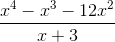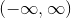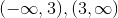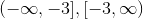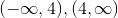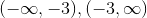Explanation:

The function in the numerator factors to: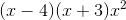so if we cancel the x+3 in the numerator and denominator we have the same function but it is continuous. The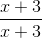gives us a hole at x=-3 so our function is not continuous at x=-3.

### Example Question #1 : Determine If A Function Is Continuous Using Limits

What are the discontinuities in the following function and what are their types?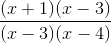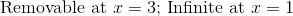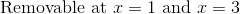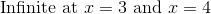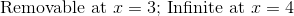Explanation:

Since the factor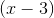is in the numerator and the denominator, there is a removable discontinuity at.  The function is not defined at, but function would move towards the same point for the resultant function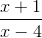.

Since the factor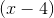cannot be factored out, there is an infinite discontinuity at.  The denominator will get very small and the numerator will move toward a fixed value.

There is no discontinuity at all at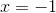.  The function simply evaluates to zero at this point.

### Example Question #1 : Determine If A Function Is Continuous Using Limits

Determine if the function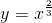is continuous atusing limits.

No, it is not continuous because the lefthand limit does not match the righthand limit.

Yes, it is continuous because the righthand and lefthand limits are equal to the actual value of the function.

No, it is not continuous because the lefthand and righthand limits do not equal the value of the function at 0.

Yes, it is continuous because the lefthand and righthand limits are equal.

Yes, it is continuous because the righthand and lefthand limits are equal to the actual value of the function.

Explanation:

In order to determine if a function is continuous at a point three things must happen.

1) Taking the limit from the lefthand side of the function towards a specific point exists.

2) Taking the limit from the righthand side of the function towards a specific point exists.

3) The limits from 1) and 2) are equal and equal the value of the original function at the specific point in question.

In our case,

1)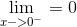2)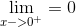3)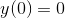Because all of these conditions are met, the function is continuous at 0.

### Example Question #45 : Introductory Calculus

Determine if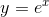is continuous on all points of its domain.

Yes, it is continuous because the righthand and lefthand limits are equal to the actual values of the function.

No, it is not continuous because the lefthand limit does not match the righthand limit.

No, it is not continuous because the lefthand and righthand limits do not equal the value of the function at 0.

Yes, it is continuous because the lefthand and righthand limits are equal.

Yes, it is continuous because the righthand and lefthand limits are equal to the actual values of the function.

Explanation:

First, find that at any point where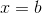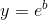.

Then find that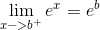and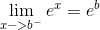.

As these are all equal, it can be determined that the function is continuous on all points of its domain.

### Example Question #1 : Determine If A Function Is Continuous Using Limits

Let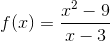. Determine if the function is continuous using limits.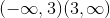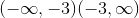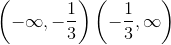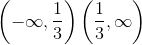Explanation:

Asapproaches, the functionapproaches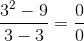, which is undefined. However, if we factor, we get: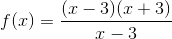Thefactors in the numerator and denominator cancel out, leaving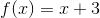.

Therefore, our function is continuous at all values offrom.

### All Precalculus Resources